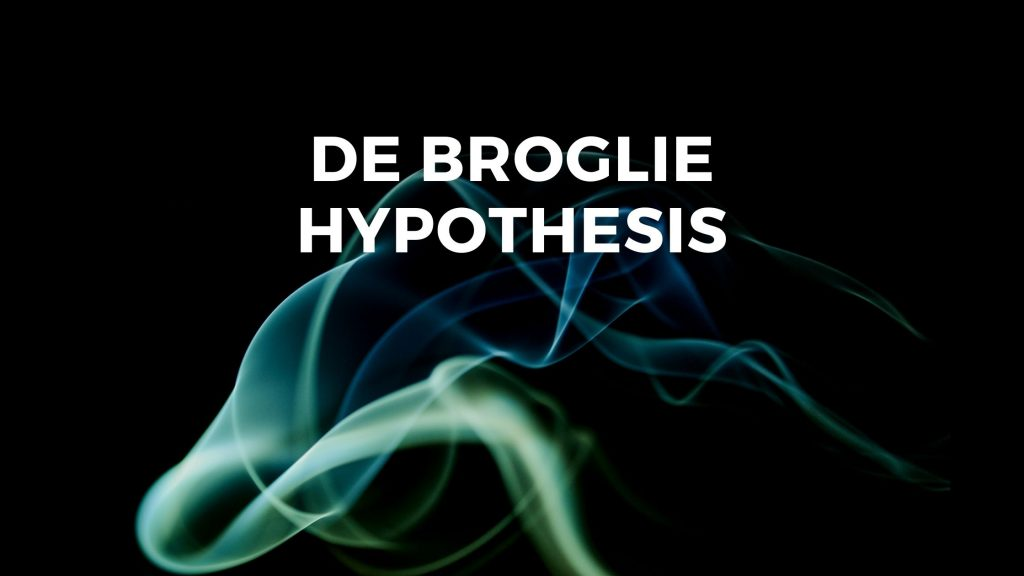Physics
Study Material

# de Broglie Hypothesis- Wave Nature of Matter

This blog contains de Broglie hypothesis and the mathematical equation. The experiment which proves de Broglie hypothesis is also covered here.

3 minutes long
Posted by Mahak Jain, 22/7/2021Hesap Oluştur

Got stuck on homework? Get your step-by-step solutions from real tutors in minutes! 24/7. Unlimited.

If we know that waves exhibit particle behavior, then a question arises in our mind that does particles exhibit wave nature? To answer the question there came Louis Victor de Broglie with de Broglie’s hypothesis. Particle nature of wave is shown by phenomenon of superposition. If the wave shows particle nature than by symmetry particles should show wave nature.

## de Broglie Hypothesis

de Broglie put a bold hypothesis, which states that moving particles of matter should display wave like properties under suitable conditions. He reasoned that nature was symmetrical and that the two basic physical entities – matter and energy, must have symmetrical character. If radiation shows dual aspects, so should matter.

## de Broglie Equation of Wavelength

de Broglie equation gives relation between momentum of particle and wavelength of wave associated with it.

λ = h/p = h/mv

This is de Broglie’s relation and λ is de Broglie wavelength.

h is Plank’s constant with value 6.626 x 10-34 Js.

Let’s take this equation a step forward.

Relation between energy and velocity of moving particle is E = p2/2m

⇒ p = √(2Em)

h = λ√(2Em)

Thus, λ = h / √(2Em), this is for particle.

## Davisson And Germer Experiment

### Significance of Davisson And Germer Experiment

This experiment physically verifies the wave nature of particles. This experiment was done on electron.

### The Experiment

The figure below shows the experimental arrangement used by Davisson and Germer.

The setup consists of, firstly an electron gun which comprises of a tungsten filament F, coated with barium oxide and heated by a low voltage power supply (L.T. or battery). Moreover, electrons emitted by the filament accelerates to a desired velocity by applying suitable potential/voltage from a high voltage power supply.

The experiment was performed by varying the accelarating voltage from 44 V to 68 V. Researchers noticed that a strong peak appeared in the intensity (I) of the scattered electron for an accelerating voltage of 54V at a scattering angle θ = 50°.

The appearance of the peak in a particular direction is due to the constructive interference of electrons scattered from different layers of the regularly spaced atoms of the crystals. From the electron diffraction measurements, the wavelength of matter waves was found to be 0.165 nm.

For V = 54V

λ = h/p = 1.227/√54 nm = 0.167 nm.

Thus, there is an excellent agreement between the theoretical value and the experimentally obtained value of de Broglie wavelength. Davisson and Germer experiment thus strikingly confirms the wave nature of electrons and the de Broglie relation.